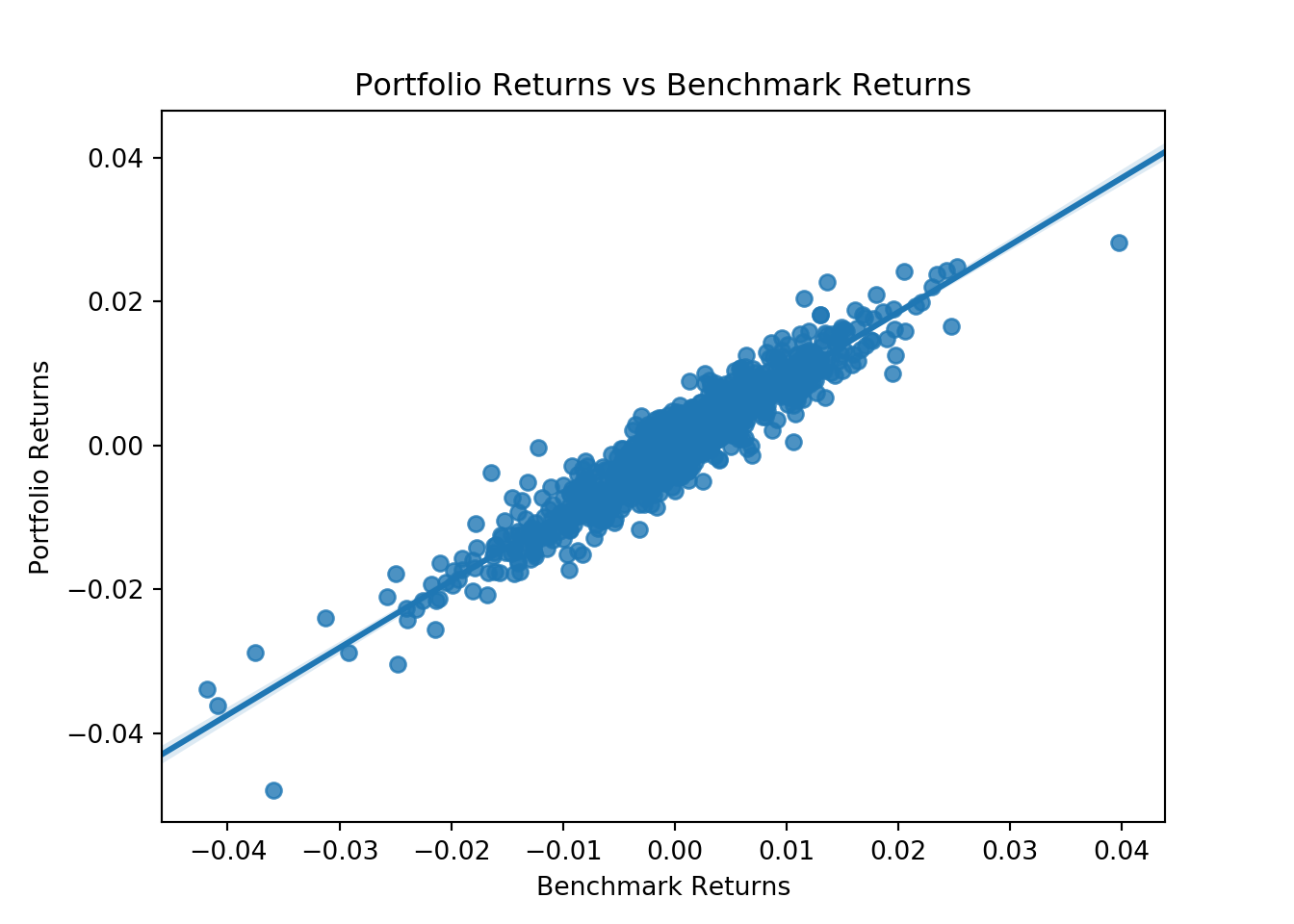In this post we will calculate the portfolio beta

``````import pandas as pd
import numpy as np
import matplotlib.pyplot as plt
import pandas_datareader as web
from scipy import stats
import seaborn as sns``````

We will use the same assets from the last post to build our portfolio.

``````# Create a list of tickers and weights
tickers = ['BND', 'VB', 'VEA', 'VOO', 'VWO']
wts = [0.1,0.2,0.25,0.25,0.2]``````

Next lets download the price data from yahoo finance.

``````price_data = web.get_data_yahoo(tickers,
start = '2013-01-01',
end = '2018-03-01')
price_data = price_data['Adj Close']``````

Then we will calculate the daily returns for our assets.

``ret_data = price_data.pct_change()[1:]``

After this we will calculate the portfolio returns.

``port_ret = (ret_data * wts).sum(axis = 1)``

In order to calculate the portfolio beta, we need to regress the portfolio returns against the benchmark returns. To do that we will use S&P 500 etf as our benchmark and calculate its returns.

``````benchmark_price = web.get_data_yahoo('SPY',
start = '2013-01-01',
end = '2018-03-01')

benchmark_ret = benchmark_price["Adj Close"].pct_change()[1:]``````

Once we have the benchmark returns, we can visualize the scatter plot of our portfolio returns versus benchmark returns.

``````sns.regplot(benchmark_ret.values,
port_ret.values)
plt.xlabel("Benchmark Returns")
plt.ylabel("Portfolio Returns")
plt.title("Portfolio Returns vs Benchmark Returns")
plt.show()``````We can see that our portfolio returns are highly correlated to the benchmark returns. We can use the regression model to calculate the portfolio beta and the portfolio alpha. We will us the linear regression model to calculate the alpha and the beta.

``````(beta, alpha) = stats.linregress(benchmark_ret.values,
port_ret.values)[0:2]

print("The portfolio beta is", round(beta, 4))``````
``## The portfolio beta is 0.9329``
``print("The portfolio beta is", round(alpha,5))``
``## The portfolio beta is -0.00016``

We can see that this portfolio had a negative alpha. The portfolio beta was 0.93. This suggests that for every +1% move in the S&P 500 our portfolio will go up 0.93% in value.